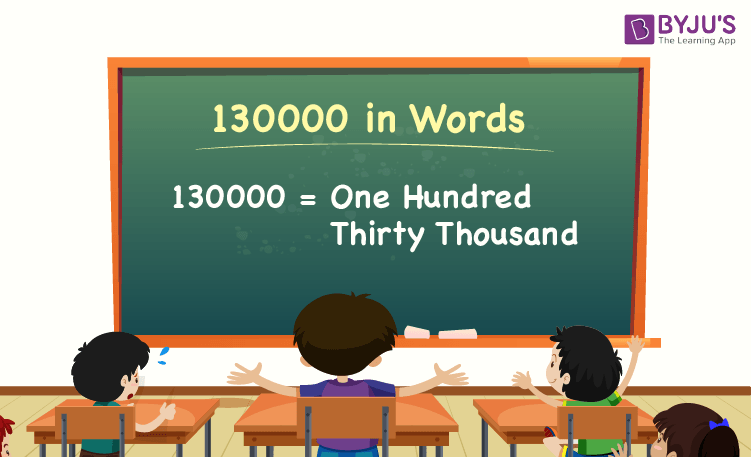# 130000 in Words

130000 in words is One Hundred Thirty Thousand. For example, if you have saved 130000 in 2 months, then you can write, “I have saved one hundred thirty thousand in two months”. We can easily write the name of numbers using a place value of a number, such as ones, tens, hundreds, thousands, etc.

 130000 in Words: One Hundred Thirty Thousand

In this article, we will learn how to write 130000 in words, i.e., the spelling of 130000 in words, and look at many solved examples.

## How to Write 130000 in Words?The place value chart helps to write the number 130000 in words. Using the ones, tens, hundreds, and thousands place value of each digit in the number 130000, we can easily write the number name of 130000.

For a number 130000,

The digit in one’s place = 0

The digit in ten’s place = 0

The digit in the hundred’s place = 0

The digit in the thousand’s place = 130

Thus, 130000 is represented in the place value chart as follows:

 Thousands Hundreds Tens Ones 130 0 0 0

Therefore, the number 130000 in words is One Hundred Thirty Thousand.

### Examples

Example 1:

Determine the value of 100000 + 30000. Express the value in words.

Solution:

Given expression: 100000 + 30000

⇒ 100000 + 30000 = 130000

So, the value of 100000 + 30000 is 130000.

Hence, 130000 in words is one hundred thirty thousand.

Example 2:

What is the value of one hundred thirty thousand minus thirty thousand in words?

Solution:

One hundred thirty thousand = 130000

Thirty thousand = 30000

One hundred thirty thousand minus thirty thousand = 130000 – 30000 = 100000

Hence, the value of one hundred thirty thousand minus thirty thousand is one hundred thousand in words.

## Frequently Asked Questions on 130000 in Words

Q1

### Write 130000 in words.

130000 in words is one hundred thirty thousand.

Q2

### What is the value of 115000 + 15000? Express the value in words.

Simplifying 115000 + 15000, we get 130000. Hence, 130000 in words is one hundred thirty thousand

Q3

### How to write one hundred thirty thousand in numbers

In numbers, One hundred thirty thousand is written as 130000.

Q4

### Is 130000 an odd number?

No, 130000 is not an odd number.

Q5

### Is 130000 a composite number?

Yes, 130000 is a composite number.

Test your Knowledge on 130000 in Words